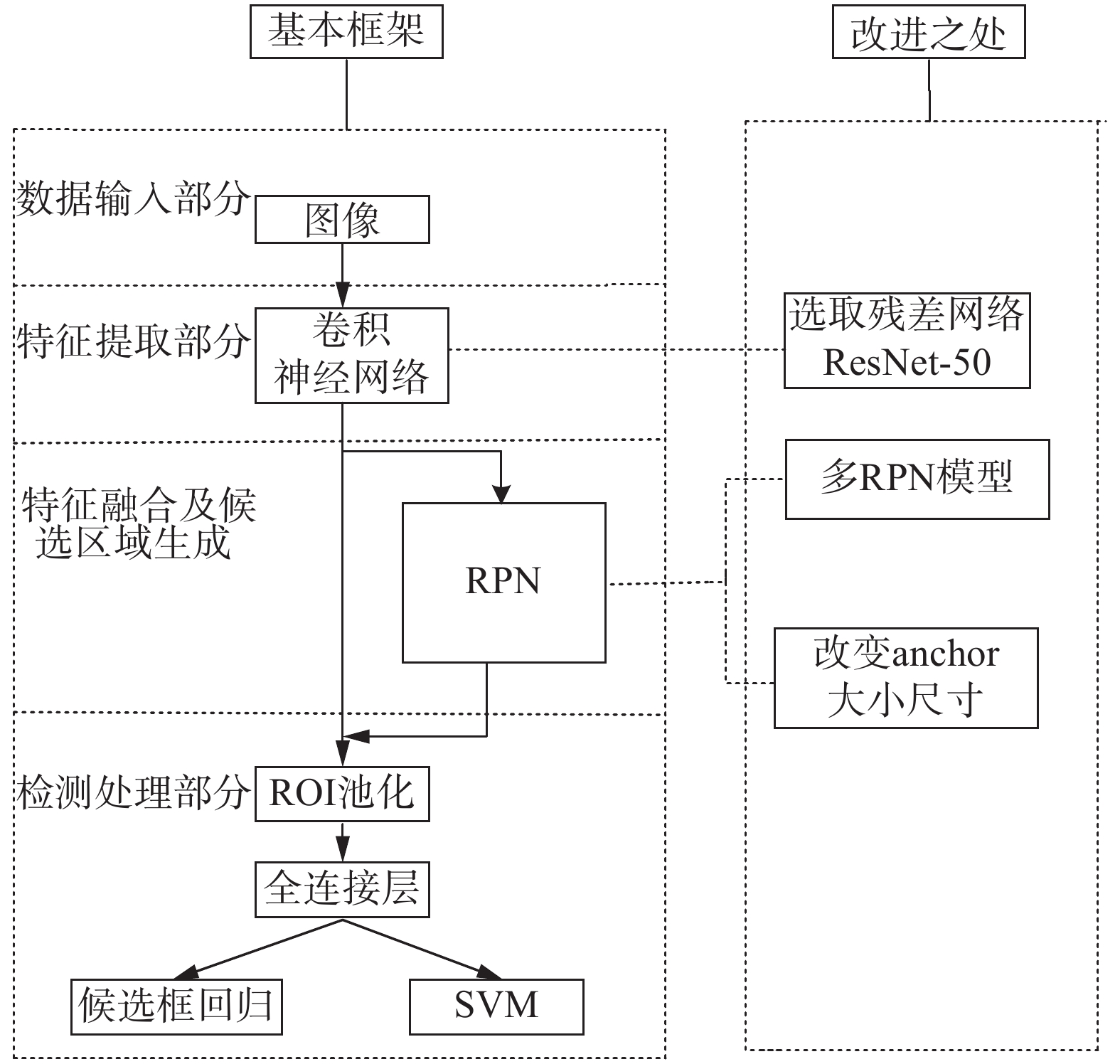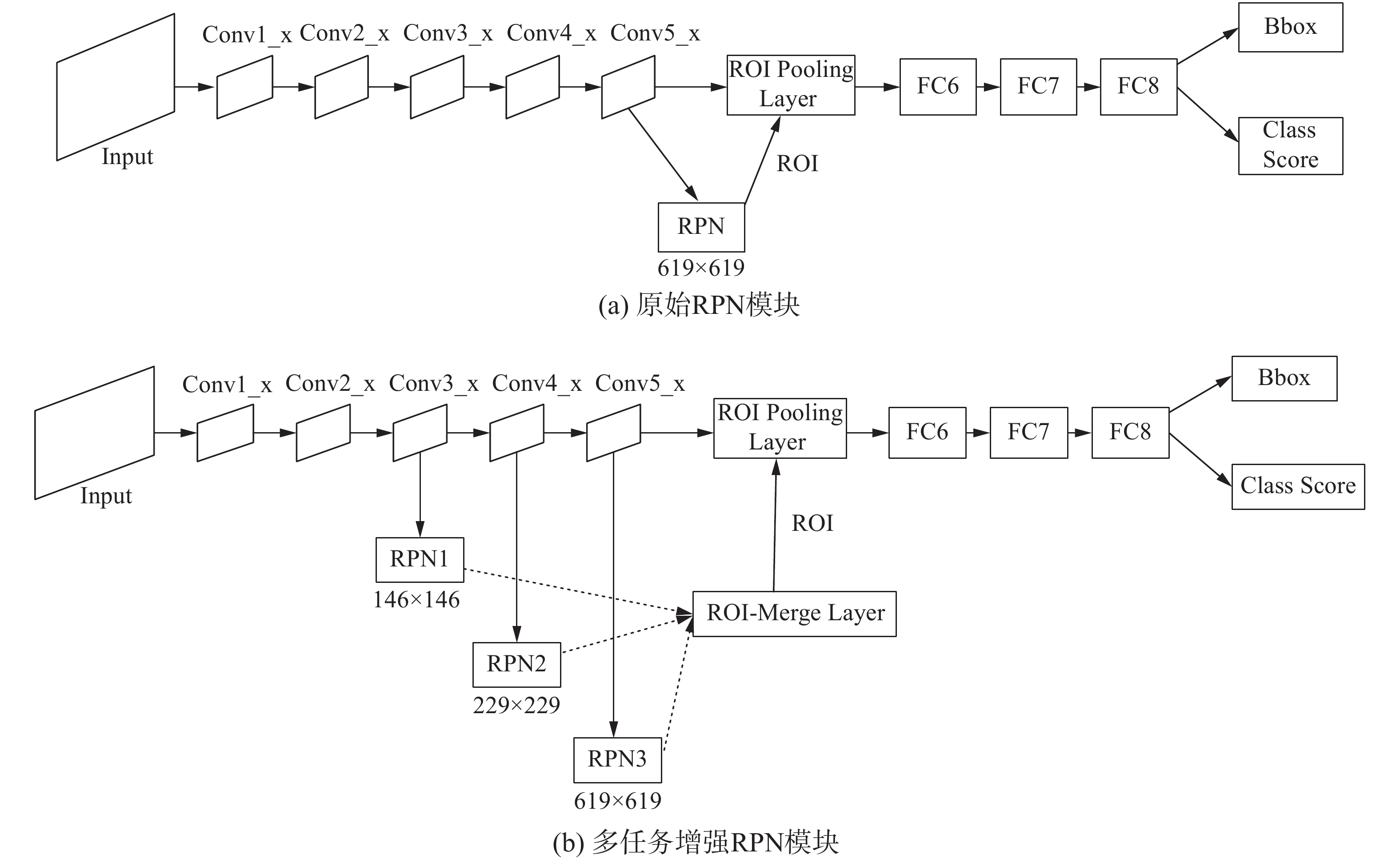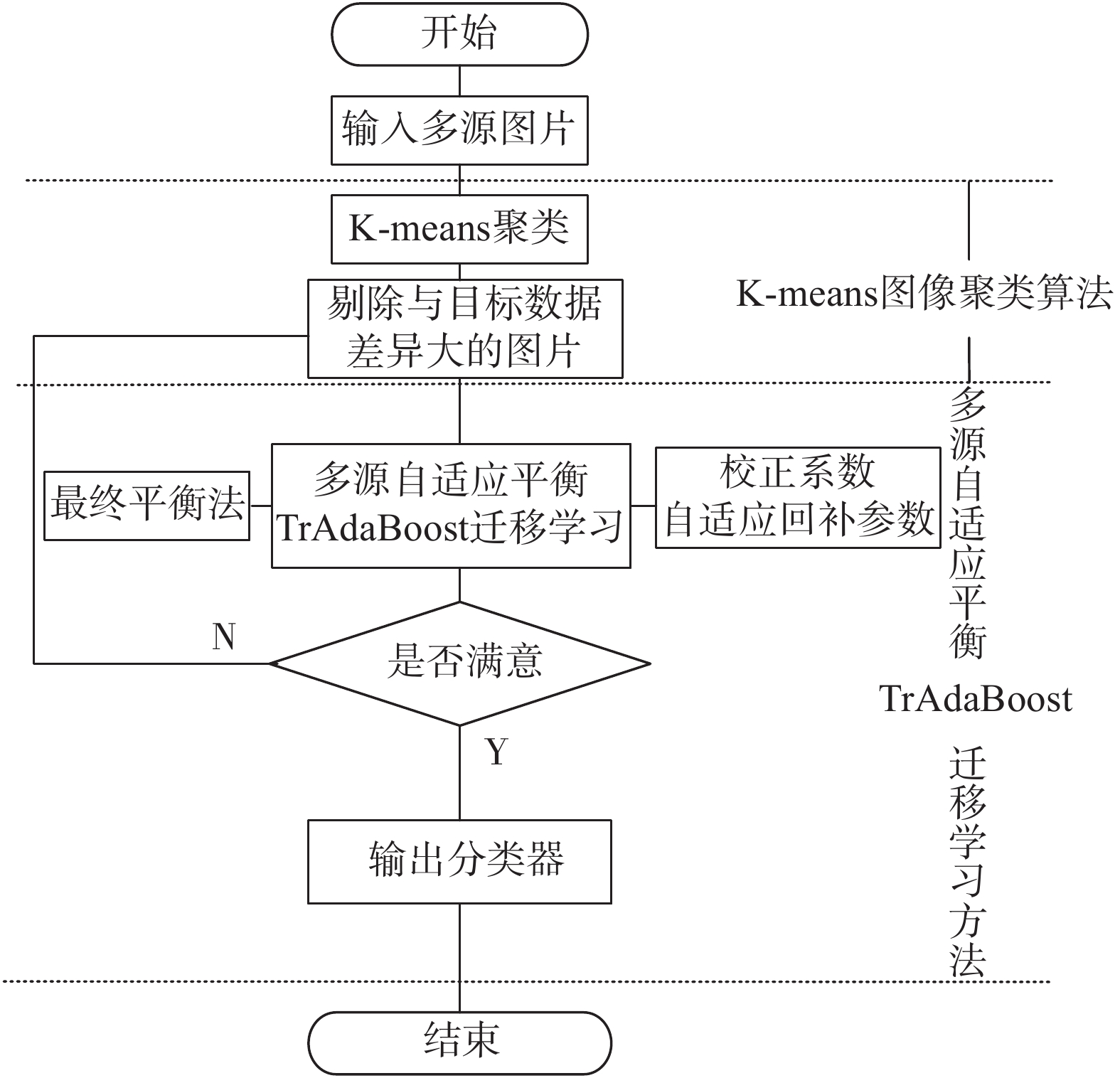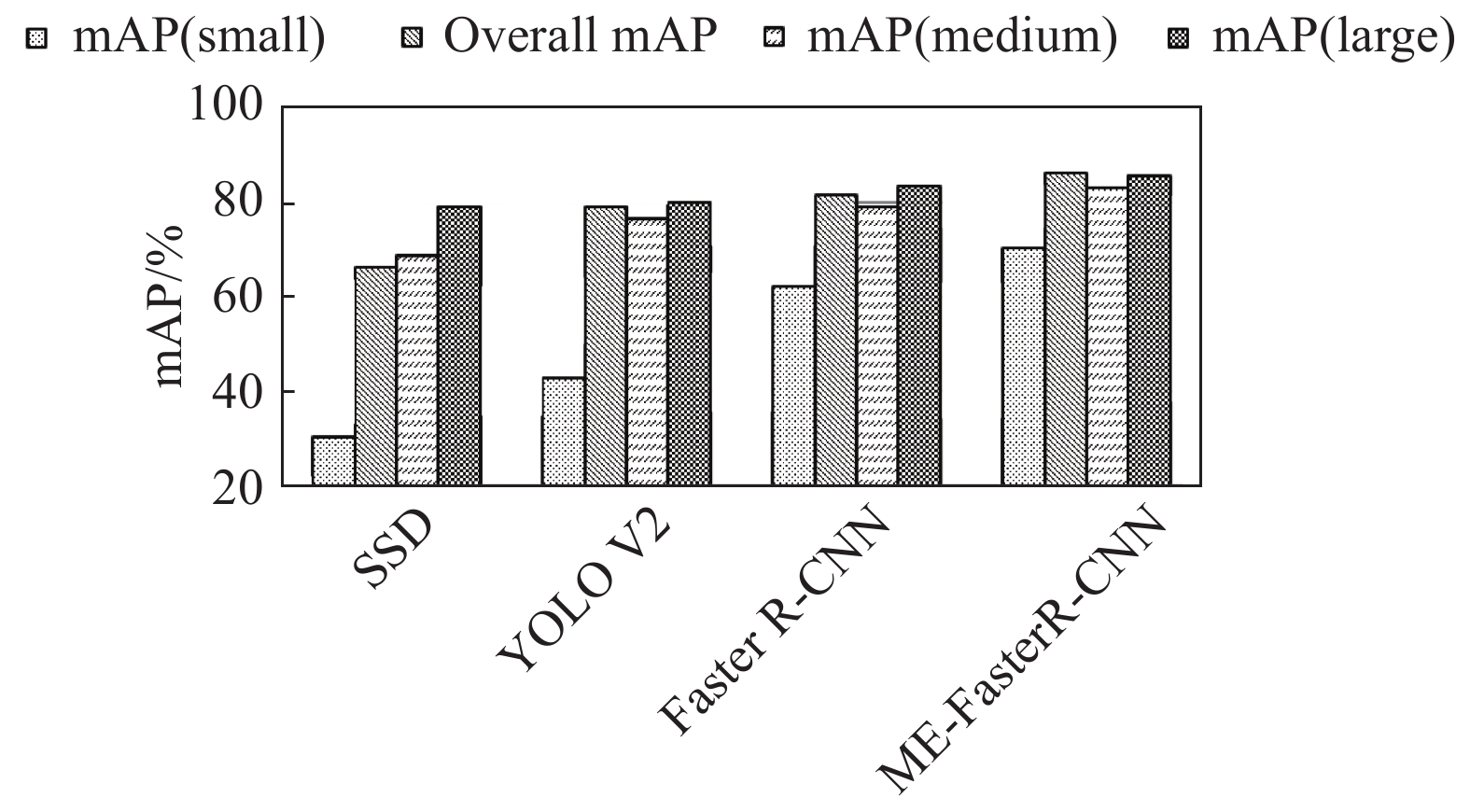﻿ 基于Faster R-CNN的多任务增强裂缝图像检测方法
«上一篇文章快速检索 高级检索

 智能系统学报2021, Vol. 16Issue (2): 286-293  DOI: 10.11992/tis.2019100040

### 引用本文MAO Yingchi, TANG Jianghong, WANG Jing, et al. Multi-task enhanced dam crack image detection based on Faster R-CNN[J]. CAAI Transactions on Intelligent Systems, 2021, 16(2): 286-293. DOI: 10.11992/tis.201910004.### 文章历史

Multi-task enhanced dam crack image detection based on Faster R-CNN
MAO Yingchi , TANG Jianghong, WANG Jing , PING Ping , WANG Longbao
College of Computer and Information, Hohai University, Nanjing 211100, China
Abstract: To improve the accuracy of the detection of multiple small targets using the faster R-CNN model, we propose a multi-task enhanced dam-crack image detection method based on faster R-CNN (ME-Faster R-CNN). In addition, to solve the problem of insufficient dam-crack samples, we propose a transfer learning method, multi-source adaptive balance TrAdaBoost based on K-means (K-MABtrA), to assist with network training. In the ME-Faster R-CNN, the ResNet-50 network is adopted to extract features from original images, obtain the feature map, and input a multi-task enhanced region-proposal-network module to generate candidate regions by adopting the appropriate size and dimensions of the anchor box. Lastly, the features map and candidate regions are processed to detect dam cracks. The K-MABtrA method first uses K-means clustering to delete unsuitable images. Then, models are trained using the multi-source adaptive balance TrAdaBoost method. Our experimental results show that the proposed ME Faster R-CNN with the K-MABtrA method can obtain an 82.52% average intersection over union (IoU) and 80.02% mean average precision (mAP). Compared with Faster R-CNN detection method using the same parameters, the average IoU and mAP values was increased by 1.06% and 1.56%, respectively.
Key words: crack image detection    Faster R-CNN    Multi-task detection    small targets detection    transfer learning    dam safety    RPN    small sample

1 相关工作

2 ME-Faster R-CNN与K-MABtrA方法

2.1 ME-Faster R-CNN模型

ME-Faster R-CNN在Faster R-CNN模型基础上进行改进，改进之处如图1所示。其中，特征提取部分：选取轻量级的ResNet-50作为卷积神经网络；特征融合部分以及候选区域生成部分：改进使用多任务增强RPN模型，改善锚盒尺寸大小提高Faster R-CNN搜索能力，提高检测识别精度；检测处理部分：特征图和选择区域建议经过感兴趣区域(ROI)池、全连接(FC)层分别发送给边界回归器和SVM分类器得到分类与回归结果。Download: 图 1 ME-Faster R-CNN模型改进之处 Fig. 1 Improvements of ME-Faster R-CNN

ME-Faster R-CNN检测流程主要分为3个部分，分别是特征提取、特征融合以及候选区域生成、检测处理。

1)特征提取：本文选用ResNet-50深度残差网络作为大坝裂缝图像特征提取器，通过5级ResNet-50将图片转换成特征图。

2)特征融合以及候选区域生成：将所得特征图输入多任务增强RPN模型，并改善RPN模型的锚盒尺寸和大小以提高检测识别精度，最后生成候选框。具体方法如下：

①多任务增强RPN方法：最初Faster R-CNN模型结构中只有一个RPN，RPN使用最后一个卷积层获得特征图。称之为原始RPN，其结构如图2(a)所示，输入图像大小为 $224 \times 224$ ，原始RPN在网络中感受野要远远大于 $224 \times 224$ ，仅能获得少量典型裂缝特征。然而，图像中裂缝存在不同大小和比例。如果检测到裂缝大小对于检测区域太大，则检测区域周围多余裂缝形状可能会被视为噪音。如果检测到裂缝大小对于检测区域太小，RPN将无法生成ROI。因此，原始RPN的功能不足以检测不同大小和比例的完整裂缝对象。Download: 图 2 原始RPN模型与多任务增强RPN模型 Fig. 2 Primitive RPN module &multi-task enhanced RPN module

②改善RPN模型的锚盒尺寸和大小：Faster R-CNN模型经过卷积层提取特征图，然后特征图输入RPN区域进行特征融合以及生成候选区域，此时特征图上每个像素点映射不同比例宽度锚点，每个锚点放置若干个不同大小的锚，为解决不同尺度间隔的锚盒搜索能力不平衡的问题，本文设计了一种新型锚盒，新型锚盒尺度为： $50 \times 50$ $200 \times 200$ $350 \times 350$ $500 \times 500$ ，其中， $50 \times 50$ $200 \times 200$ 适用于较小的裂缝检测， $350 \times 350$ $500 \times 500$ 适用于较大的裂缝检测。这4种尺度每种尺寸按1∶1，1∶2，2∶1 的长宽比例缩放，共12种尺度作为RPN需要评估的候选框，在预测时候选框的顺序是固定的。RPN的目标就是对原图中的每个锚点对应的12个框，预测其是否是一个存在目标的框。框与真值框的 ${\rm{IoU}} > 0.7$ 就认为这个框是一个候选框，反之，则不是。

2.2 K-MABtrA方法

1) K-means图像聚类方法：通过K-means图像聚类方法，利用欧式距离将图像进行聚类排序。将聚类距离远的图片从裂缝图像库中删除，有利于后续分类器的训练，提高训练效率。K代表聚类质心数目，means表示簇内数据的均值。K-means图像聚类方法具体步骤如下：

①首先将图像库中图像 ${X_i}(i = 1,2, \cdots ,n)$ 进行灰度化，依次存储到一维矩阵 ${{{D}}_X}$ 中；

②接着以10像素长度，3像素移动步长依次进行分块存储，记录每小块的首位置，得到n个像素块数据集，从中任意选择30个图像小块的灰度均值作为初始聚类中心；

③根据每个图像矩阵小块的灰度均值，利用欧几里得距离，如式(1)所示，计算这些对象与30个图像样本聚类中心的距离；并根据最小距离重新对相应图像小块灰度均值进行划分，将每个图像矩阵小块赋给最相近的类；

 ${\rm{dis}}({x_i},{y_j}){\rm{ = }}{\left[ {\sum\limits_{k = 1}^d {{{({x_{ik}} - {x_{jk}})}^2}} } \right]^{\frac{1}{2}}},i,j = 1,2, \cdots ,n$ (1)

④重新计算每个有变化的图像小块像素灰度均值的质心；

⑤重复上述步骤3)、4)直至各个数据类的集合中心不再发生变化为止。Download: 图 3 K-MABtrA方法流程图 Fig. 3 Flow chart of K-MABtrA method

① 增加校正系数更新源领域样本的权值

 ${S_n} = \sum\limits_{i = 1}^{{n_a}} {{{w}}_{{a_i}}^{m + 1}} = \sum\limits_{i = 1}^{{n_a}} {{{w}}_{{a_i}}^m = {n_a}{{w}}_a^m}$ (2)

 ${S_{{b_1}}}{\rm{ = }}{{{n}}_b}{{w}}_b^m(1 - \varepsilon _b^m)$ (3)

 ${S_{{b_2}}} = {n_b}{{w}}_b^m\varepsilon _b^m\varphi _m^{ - 1} = = {n_b}{{w}}_b^m(1 - \varepsilon _b^m)$ (4)

 ${S_b} = {S_{{b_1}}} + {S_{{b_2}}} = 2{n_b}{{w}}_b^m(1 - \varepsilon _b^m)$ (5)

$m + 1$ 次迭代的辅助数据集样本权值分布为

 ${{w}}_a^{m + 1} = \dfrac{{{{w}}_a^m}}{{{S_a} + {S_b}}} = \dfrac{{{{w}}_a^m}}{{{n_a}{{w}}_a^m + 2{n_b}{{w}}_b^m(1 - \varepsilon _b^m)}}$ (6)

 ${S_a} + {S_b}{\rm{ = }}{n_a}{{w}}_a^m + 2{n_b}{{w}}_b^m(1 - \varepsilon _b^m){\rm{ = }}1$ (7)

 $\begin{array}{c} {{w}}_a^{m + 1} = {C^m}{{w}}_a^m/({C^m}{S_a} + {S_b}) =\\ {C^m}{{w}}_a^m/\left[ {{C^m}{n_a}{{w}}_a^m + 2{n_b}{{w}}_b^m(1 - \varepsilon _b^m)} \right] \end{array}$ (8)

 ${C^m} = 2(1 - \varepsilon _b^m)$ (9)

② 引入自适应回补参数

 ${B^m} = (1 - \varepsilon _{\rm{b}}^m) + (1 - \varepsilon _a^m)$ (10)

$m + 1$ 次迭代后辅助领域数据样本权值：

 $\begin{array}{c} {{w}}_a^{m + 1} = \dfrac{{{B^m}{{w}}_a^m{\alpha ^{|f({x_i}) - {y_i}|}}}}{{{S_a}{\rm{ + }}{S_b}}} = \dfrac{{{B^m}{{w}}_a^m{\alpha ^{|f({x_i}) - {y_i}|}}}}{{{{{B}}^m}{{{n}}_a}{{w}}_a^m{\rm{ + }}2{n_b}{{w}}_b^m(1 - \varepsilon _b^m)}} = \\ {B^m}{{w}}_a^m{\alpha ^{|f({x_i}) - {y_i}|}} \end{array}$ (11)

③ 最终平衡权重法

3 实验与结果 3.1 数据集介绍

3.2 实验结果与分析

1)视觉对比分析Download: 图 4 视觉对比分析 Fig. 4 Visual contrast analysis

2)与不同基准网络模型之间对比分析表 1 不同基准网络模型的准确度 Tab.1 Accuracy of different baseline network models

3)与不同目标检测算法对比分析表 2 不同目标检测算法对比分析 Tab.2 Comparison of different target detection algorithmsDownload: 图 5 不同尺寸裂缝图像的准确度 Fig. 5 Accuracy of crack images with different sizes

4)迁移学习对比实验表 3 不同迁移学习方法对比分析 Tab.3 Comparison of different transfer learning methods
4 结束语

  苏南. 我国200米级高坝密集, 安全风险不可轻视[EB/OL]. (2017-11-09).https://www.thepaper.cn/newsDetail_forward_1858088. (0)  REN Shaoqing, HE Kaiming, GIRSHICK R, et al. Faster R-CNN: towards real-time object detection with region proposal networks[C]//Proceedings of the 28th International Conference on Neural Information Processing Systems. Cambridge, USA, 2015: 91−99. (0)  HABER E, RUTHOTTO L, HOLTHAM E, et al. Learning across scales-a multiscale method for convolution neural networks[C]//Proceedings of the 23nd AAAI Conference on Artificial Intelligence. 2017. (0)  GERBER D, MEIER S, KELLERMANN W. Efficient target activity detection based on recurrent neural networks[C]//Proceedings of 2017 Hands-free Speech Communications and Microphone Arrays. San Francisco, USA, 2017: 46−50. (0)  GIRSHICK R, DONAHUE J, DARRELL T, et al. Rich feature hierarchies for accurate object detection and semantic segmentation[C]//Proceedings of 2014 IEEE Conference on Computer Vision and Pattern Recognition (CVPR). Columbus, USA, 2014: 580−587. (0)  GIRSHICK R. Fast R-CNN[C]//Proceedings of the 2015 IEEE International Conference on Computer Vision. Santiago, Chile, 2015: 1440−1448. (0)  LIU WEI, ANGUELOV D, ERHAN D, et al. SSD: single shot MultiBox detector[C]//Proceedings of the 14th European Conference on Computer Vision. Amsterdam, the Netherlands, 2016: 21−37. (0)  REDMON J, FARHADI A. YOLO9000: better, faster, stronger[C]//Proceedings of 2017 IEEE Conference on Computer Vision and Pattern Recognition. Honolulu, USA, 2017: 6517−6525. (0)  KANG H H, LEE S W, YOU S H, et al. Novel vehicle detection system based on stacked DoG kernel and AdaBoost[J]. PLoS one, 2018, 13(3): e0193733. DOI:10.1371/journal.pone.0193733 (0)  DAI Wenyuan, YANG Qiang, XUE Guirong, et al. Boosting for transfer learning[C]//Proceedings of the 24th International Conference on Machine Learning. New York, USA, 2007: 193−200. (0)  AL-STOUHI S, REDDY C K. Adaptive boosting for transfer learning using dynamic updates[C]//Joint European Conference on Machine Learning and Knowledge Discovery in Databases. Berlin, Germany, 2011: 60−75. (0)  郭勇. 基于单源及多源的迁移学习方法研究[D]. 西安: 西安电子科技大学, 2013. GUO Yong. Research of transfer learning based on single-source and multi-source[D]. Xi’an: Xidian University, 2013. (0)  HE Kaiming, ZHANG Xiangyu, REN Shaoqing, et al. Deep residual learning for image recognition[C]//Proceedings of the 2016 IEEE Conference on Computer Vision and Pattern Recognition. Las Vegas, USA, 2016: 770−778. (0)  WICAKSONO Y A, RIZALDY A, FAHRIAH S, et al. Improve image segmentation based on closed form matting using K-means clustering[C]//Proceedings of 2017 International Seminar on Application for Technology of Information and Communication. Semarang, Indonesia, 2018: 26−30. (0)  AKCAY S, KUNDEGORSKI M E, WILLCOCKS C G, et al. Using deep convolutional neural network architectures for object classification and detection within X-ray baggage security imagery[J]. IEEE transactions on information forensics and security, 2018, 13(9): 2203-2215. DOI:10.1109/TIFS.2018.2812196 (0)  RAHMAN M A, WANG Yang. Optimizing intersection-over-union in deep neural networks for image segmentation[C]//Proceedings of 12th International Symposium on Advances in Visual Computing. Las Vegas, USA, 2016: 234−244. (0)  ZEILER M D, FERGUS R. Visualizing and understanding convolutional networks[C]//Proceedings of 13th European Conference on Computer Vision. Zurich, Switzerland, 2014: 818−833. (0)  SIMONYAN K, ZISSERMAN A. Very deep convolutional networks for large-scale image recognition[C]//Proceedings of 3rd International Conference on Learning Representations. San Diego, USA, 2015. (0)